# THE TIME VALUE OF MONEYReading time: 1 minute

THE TIME VALUE OF MONEY

The time value of money is a relationship between the value of money today and its value at some future date, considering the interest charged or paid for the use of money. When an asset is purchased with the provision that the cost of asset will be liquidated over several pay periods at a specified rate of interest, it is necessary to know in advance the amount of each payment to be made. Because of the interest, the sum of the delayed payments will exceed the amount that would be paid for cash purchase.

If P rupees are the borrowed or invested at an interest rate i per period of several periods, with the earned interest to be retained and compounded at the end of each period, the future amounts at the end of the nth period will be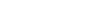Or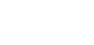The above is the formula for single payment.

The debt created by the purchase of an asset is frequently liquidated by making uniform end of period payments for the necessary number of periods.

If a uniform amount R is invested at the end of each period for n periods at compound interest i per period, the total amount at the end of n periods will be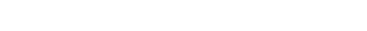From the above, it can be deduced as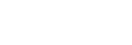This is referred to as a sinking fund formula because it determines the uniform end-of-period investment R, that must be made in order to provide the amount T at the end of n periods.

The uniform end of period payment R required to liquidate a current debt P in n periods will be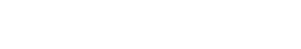Known as capital recovery formula.

The expression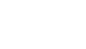is called the liquidating factor.

Tables are available for different interest rates.